TWiki>CMSPublic Web>FWD10005ExclusiveDiMuon (2012-02-25, JonathanHollar)

# FWD-10-005: Exclusive photon-photon production of muon pairs in proton-proton collisions at sqrt(s) = 7 TeV

## Abstract

A measurement of the exclusive two-photon production of muon pairs in proton-proton collisions at sqrt(s)= 7 TeV, pp to p mu^+ mu^- p, is reported using data corresponding to an integrated luminosity of 40 inverse picobarns. For muon pairs with invariant mass greater than 11.5 GeV, transverse momentum pT(mu) > 4 GeV and pseudorapidity |eta(mu)| < 2.1, a fit to the dimuon pt(mu^+ mu^-) distribution results in a measured cross section of sigma(pp to p mu^+ mu^- p) = 3.38 [+0.58 -0.55] (stat.) +/- 0.16 (syst.) +/- 0.14 (lumi.) pb, consistent with the theoretical prediction evaluated with the event generator Lpair. The ratio to the predicted cross section is 0.83 [+0.14-0.13] (stat.) +/- 0.04 (syst.) +/- 0.03 (lumi.). The characteristic distributions of the muon pairs produced via photon-photon fusion, such as the muon acoplanarity, the muon pair invariant mass and transverse momentum agree with those from the theory.

## Public CDS entry

Linkto the final paper entry in CDS.

## Approved Plots from FWD-10-005

Figure Caption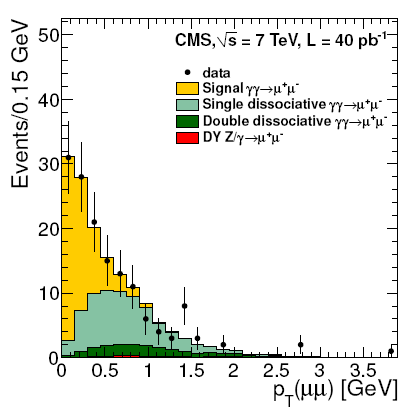Distribution of pT(mu+mu-) for the selected sample. Data are shown as points with statistical error bars. The histograms represent the simulated signal (yellow), single (light green) and double (dark green) proton dissociative backgrounds, and DY (red). The yields are determined from a fi t using the distributions from simulation.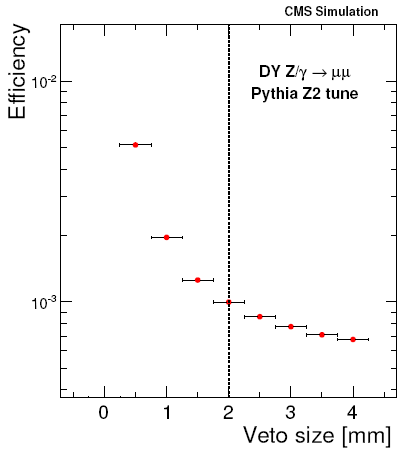Efficiency of the zero extra tracks selection vs. distance to closest track computed for DY events in simulation. Events to the right of the vertical dashed line are selected. The vertical error bars are negligible.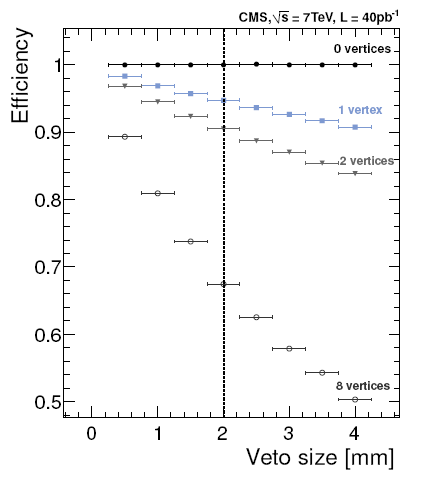Efficiency of the zero extra tracks selection vs. distance to closest track computed with the arti cial vertex method in zero-bias data. The points correspond to events with 0, 1, 2, and 8 real vertices in the event. Events to the right of the vertical dashed line are selected. The vertical error bars are negligible.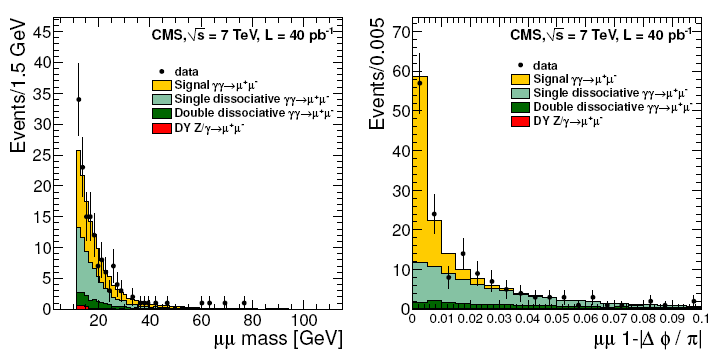Muon pair invariant mass spectrum (left) and acoplanarity (right), with all selection criteria applied and the simulation normalized to the best- t value. Data are shown as points with statistical error bars, while the histograms represent the simulated signal (yellow), single (light green) and double (dark green) proton dissociative backgrounds, and DY (red).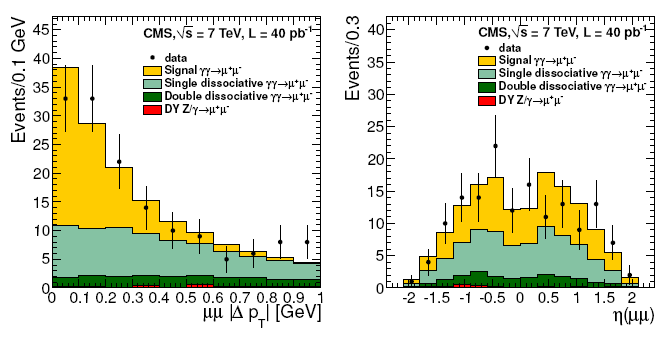Muon pair transverse momentum di erence (left) and pair pseudorapidity (right), with all selection criteria applied and the simulation normalized to the best- t value. Data are shown as points with statistical error bars, while the histograms represent the simulated signal (yellow), single (light green) and double (dark green) proton dissociative backgrounds, and DY (red).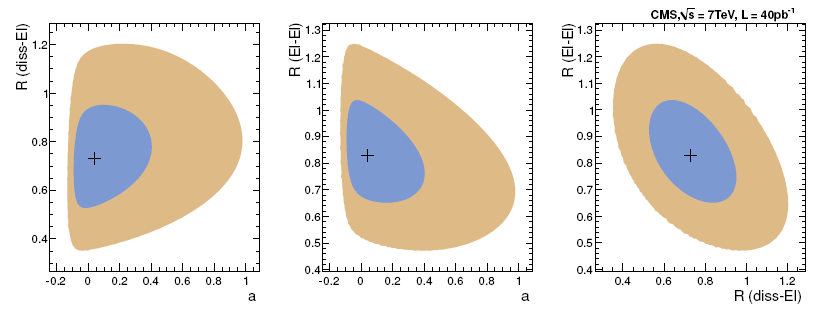One and two standard-deviation contours in the plane of tted parameters for the proton-dissociation yield ratio vs. modi cation parameter a (left), the data-theory signal ratio vs. modi cation parameter a (center), and the data-theory signal ratio vs. proton-dissociation yield ratio (right). The contours represent 39.3% and 86.5% con dence regions, where the cross indicates the best-fi t point.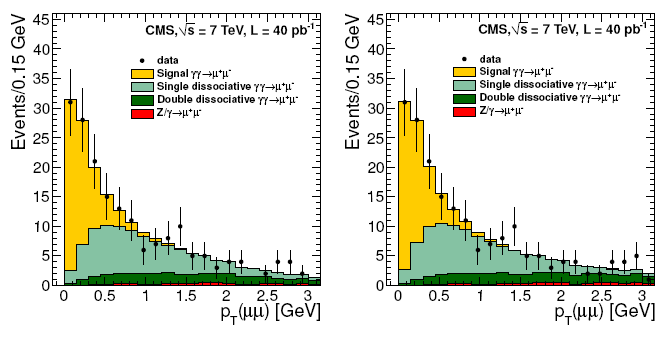Result of t to the pT(mu+mu-) distribution with requirements on delta-pT(mu+mu-) (left) and on both delta-pT(mu+mu-) and delta-phi(mu+mu-) (right) removed. The points with error bars represent the data. The histograms are the result of fitting the simulated distributions to the data.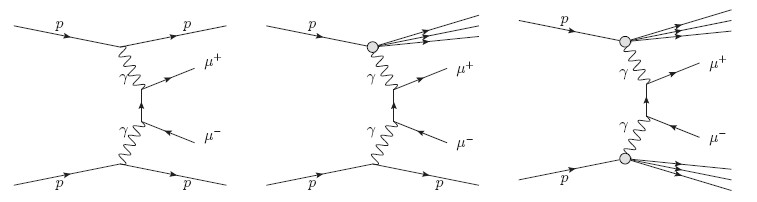Schematic diagrams for the exclusive and semi-exclusive two-photon production of muon pairs in pp collisions for the elastic (left), single dissociative (center), and double dissociative (right) cases. The three lines in the nal state of the center and right plots indicate dissociation of the proton into a low-mass system N.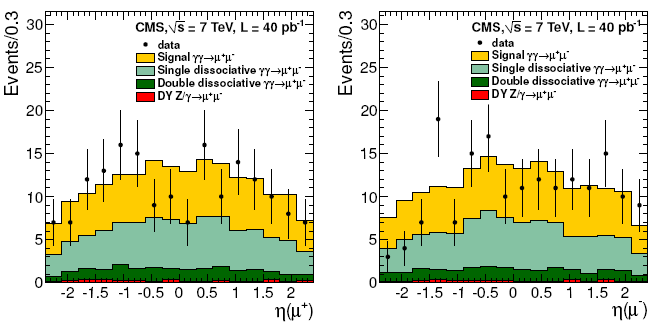Single-muon pseudorapidity distribution with all other selections applied for mu+ (left) and mu- (right) and the simulation normalized to the best- fit value. Data are shown as points with statistical error bars, while the histograms represent the simulated signal (yellow), single (light green) and double (dark green) proton dissociative backgrounds, and DY (red).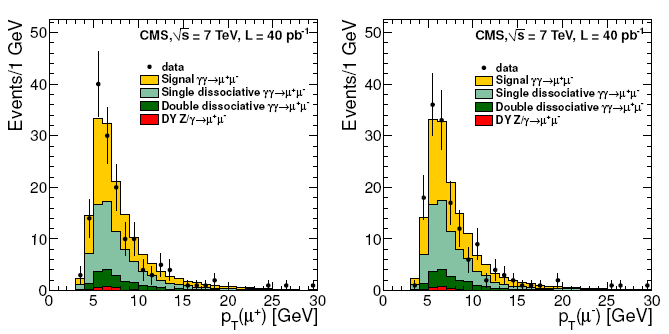Single-muon transverse momentum with all other selections applied for mu+ (left) and mu- (right) and the simulation normalized to the best-fi t value. Data are shown as points with statistical error bars, while the histograms represent the simulated signal (yellow), single (light green) and double (dark green) proton dissociative backgrounds, and DY (red).

-- JonathanHollar - 25-Feb-2012

Topic revision: r1 - 2012-02-25 - JonathanHollarLog In

Create a LeftBarCern SearchTWiki SearchGoogle Search CMSPublic All websCopyright &© 2008-2020 by the contributing authors. All material on this collaboration platform is the property of the contributing authors.
Ideas, requests, problems regarding TWiki? Send feedback